# Fog

The car started in fog at a speed of 30 km/h. After a 12-minute drive, the fog dissipated, and the driver drove the next 12 minutes distance, 17 km. On the last 17 km long again, the driving conditions deteriorated, and the driver drove at a speed of 51 km/h. a) Calculate the distance of the first section, the speed on the second section, and the time on the third section. b) Construct the graph distance vs. time t. c) Calculate the average speed for the first two sections and the average speed on the last two sections. When can the average rate calculated as the arithmetic mean of the individual speeds? Judge answer.

s1 =  6 km
v2 =  85 km/h
t3 =  0.3333 h
c1 =  57.5 km/h
c2 =  63.75 km/h

### Step-by-step explanation: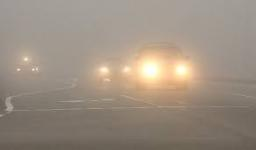Did you find an error or inaccuracy? Feel free to write us. Thank you!Tips to related online calculators
Looking for help with calculating arithmetic mean?
Looking for a statistical calculator?
Do you want to convert velocity (speed) units?
Do you want to convert time units like minutes to seconds?

#### You need to know the following knowledge to solve this word math problem:

We encourage you to watch this tutorial video on this math problem:

## Related math problems and questions:

• Constant motionThe car has undergone constant motion 120 km in 1 hour 30 minutes. Determine its average speed. Determine how long the car drove in the first 20 minutes and 50 minutes from the start of the move.
• DriverThe driver of the supply car reckoned that at the average speed of 72 km/h arrives to the warehouse for 1 1/4 hours. After 30 km however unintentionally drove to the gas station and have ten minutes delay. At what average speed would have to go the rest o
• Motion 2At 10 o'clock started from place A car speed at 90 km/h. Twenty minutes after starting another car from place B at a speed 60 km/h . Distance places A and B is 120 kilometers. What time and where do they meet?
• Average speed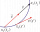The car drove on one section of the highway for half an hour at a speed of 80 km/h. Then he reduced his speed to 60km/h. It went three-quarters of an hour at a speed of 60 km/h. What was the average speed of the car?
• MotionFrom two different locations distant 232 km started against car and bus. The car started at 5:20 with average speed 64 km/h. Bus started at 7:30 with average speed 80 km/h. When they meet? How many kilometers went the bus?
• Two citiesThe distance between cities A and B is 132 km. At 9.00 am, the cyclist started the bike at an average speed of 24 km/h, and at 10.00 am started from the B cyclist at an average speed of 30 km/h. How long and far from A will they both meet?
• Warehouse carsFrom the warehouse started truck at speed 40km/h. After 1hour 30mins started from the same place same direction a car at speed 70 km/h. For how long and at what distance from the warehouse overtake a truck?
• Cyclist vs car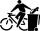Cyclist rode out of the city at 18 km/h. 1 hour 30 minutes behind him started car and caught up with the cyclist in 50 minutes. How fast was driving the car? Where (what kilometer) from the city car overtook a cyclist?
• Car and cyclist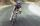Cyclist started from town at a speed of 18 km/h. After 1 hour 30 min started the car from town and caught up with the cyclist in 50 minutes. How fast was a car?
• Peleton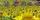The cycling race was run at an average speed of 45 km/h. One cyclist lost with defect 8 minutes. How long and how far he must go at speed 51 km/h to catch the peloton again?
• Sebastian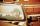From Kovalov Sebastian walks at 6 km / h started at 8:00 in the direction of Kuty. From Kuty, the godfather is driving at 50 km/h and started at 8:30. The distance is 24 km. When and where grandfather will take Sebastian to the car.
• Two trains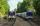Two trains go at each other. First at speed 40 km/h and the second at speed 60 km/h. At what distance will be 30 minutes before they will meet?
• Car driver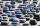The car driver is in town A and is scheduled to be in town B at a specified hour. If it travels at an average speed of 50km/h, it will arrive in city B 30 minutes later. But if he travels at an average speed of 70km/h, he would arrive half an hour earlier
• Znojmo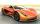From Znojmo to Brno started the truck with a trailer at an average speed 53 km per hour. Against him, 14 minutes later from Brno started the car with an average speed of 1.2-times greater than the truck. How long and how far from Znojmo, they meet if the
• Average speedThe car drove from city A to city B at a 40 km/h and back to city A at a speed of 80 km/h. What was the average speed of the car?
• Two citiesThe car goes from city A to city B at an average speed of 70 km/h, back at an average speed of 50 km/h. If it goes to B and back at an average speed of 60 km/h, the whole ride will take 8 minutes less. What is the distance between cities A and B?
• Tourists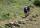From the cottage started first group of tourists at 7:00 AM at speed 4 km/h. The second started after them 21 minutes later at speed 6 km/h. How long and how many kilometers from the cottage will catch the first group?